### Home > CAAC > Chapter 2 > Lesson 2.1.3 > Problem2-33

2-33.
1. Without a calculator, compute the value of each expression below. Homework Help ✎

1. −14 + (−31)

2. −(−8) − (−2)

3.4. −11 · 24

5.6. 46 ÷ (−23)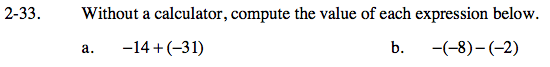If you drew the tiles, would you have any zero pairs?

−14 + (−31)= −45

Change the subtraction into addition to eliminate excess negatives and then try solving using the same system used in part (a).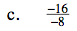When dividing or multiplying with negatives, proceednormally and account for negatives afterward.

When dividing or multiplying with negative numbers, if there are an even number of negatives then the answer is positive; if there are an odd number of negatives then the answer too is negative.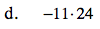Try ignoring the negative signs to start and multiply as normal. At the end, account for any negative signs.

−264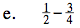Find a common denominator for the fractions in order to subtract.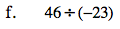Remember that since you are dividing a positive number by a negative number, your answer will be negative.

If you are having difficulty, try making all of the subtraction problems into addition problems.